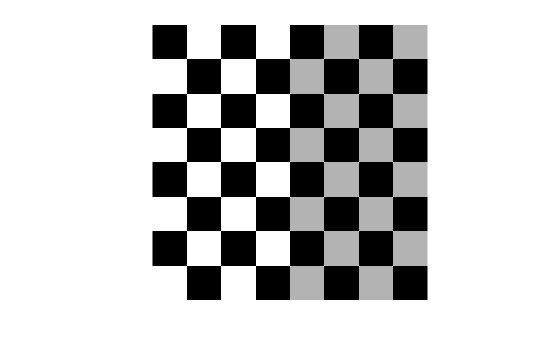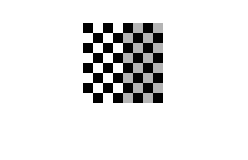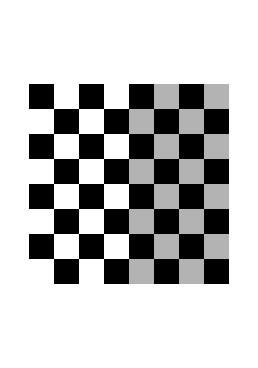# truesize

## Syntax

``truesize(fig,[mrows ncols])``
``truesize(fig)``

## Description

example

````truesize(fig,[mrows ncols])` adjusts the display size of an image in a figure, `fig`, to the dimensions `[mrows ncols]`, in pixels.```
````truesize(fig)` adjusts the display size such that each image pixel covers one screen pixel.If you do not specify a figure, `truesize` adjusts the display size of the current figure.```

## Examples

collapse all

Create a default checkerboard image, which has size 80-by-80 pixels. Display the checkerboard image to fill the full size of the figure window. The image is magnified to fill the window.

```c = checkerboard; imshow(c,'InitialMagnification','fit')```Display the checkerboard image so that each image pixel covers one screen pixel.

`truesize`You can adjust the size of the figure window to arbitrary dimension. The image scales to fit within the figure window.

`truesize([300 200]);`## Input Arguments

collapse all

Figure containing a single image or a single image with a color bar, specified as a figure handle. By default, `truesize` uses the current figure, with handle `gcf`.

Screen dimensions (in pixels) that the image should occupy, specified as a 2-element numeric row vector. By default, `[mrows ncols]` is equal to the image size, so each image pixel covers one screen pixel.

## Version History

Introduced before R2006a